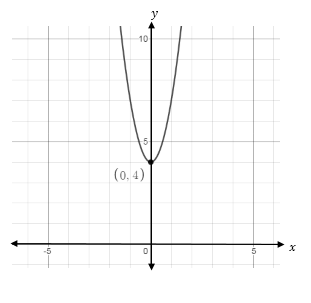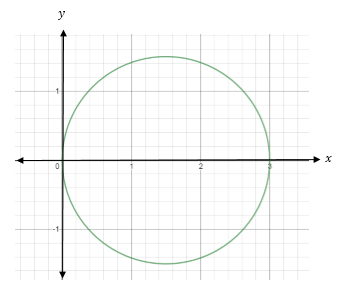# a) Eliminate the parameter to find a Cartesian equation of the curve. x(t) = \sqrt{t}, y(t) = 3t...

## Question:

a) Eliminate the parameter to find a Cartesian equation of the curve.

$$x(t) = \sqrt{t}, \, y(t) = 3t + 4, \, t \geq 0.$$

Graph the resulting equation.

b) Find the equations of the tangent and normal lines at the point {eq}t = 1 {/eq} on the curve {eq}x(t) = 2t {/eq} and {eq}y(t) = t^{2} - 1 {/eq}.

c) Graph the following parametric equations

$$r = 3 \cos(\theta) \text{ for } \frac{\pi}{2} \, \leq \theta \leq \frac{3 \pi}{2}$$

## Parametric to Cartesian Equation Conversion:

Parametric equations are those where two are dependent and one independent variable present.

To convert parametric to Cartesian equation we have to eliminate independent variable between two dependent variable and find the relation between that two dependent variable as one is dependent and other independent.

Tangent line is the line on a graph which touches the curve at only one point.

And normal line is the line perpendicular to the curve at only one point.

Give information is

(a)

The parametric equations of curve are

{eq}\displaystyle x(t) = \sqrt{t}, \, y(t) = 3t + 4, \, t \geq 0.\\ \displaystyle i.e\:t=x^2 \displaystyle \therefore\:y=3x^2+4 {/eq}

Which is Cartesian form equation.

Graph :(b)

Given parametric equations are

{eq}\displaystyle x(t) = 2t {/eq} and {eq}\displaystyle y(t) = t^{2} - 1 {/eq}.

At t=1

x=2 and y=0

Let's find Cartesian equation of them

{eq}\displaystyle \:t=\frac{x}{2}\\ \displaystyle \therefore y=\left(\frac{x}{2}\right)^2-1\:\\ \displaystyle i.e\:y=\frac{x^2}{4}-1 {/eq}

Let's find tangent and normal equations.

Here,

Slope of tangent {eq}\displaystyle m=y'=\frac{x}{2}\\ \displaystyle at\:x=2\\ \displaystyle m=1 {/eq}

As slope of normal is negative reciprocal of slope of tangent,

slope of normal line {eq}\displaystyle m_1\:=-1 {/eq}

Hence, equation of tangent line at point (2,0) by point slope form is

{eq}\displaystyle y-0=1\left(x-2\right)\\ \displaystyle y=x-2 {/eq}

And equation of normal is

{eq}\displaystyle \:\:y-0=-1\left(x-2\right)\\ \displaystyle y=2-x {/eq}

(c)

Graph

{eq}\displaystyle r = 3 \cos(\theta) \text{ for } \frac{\pi}{2} \, \leq \theta \leq \frac{3 \pi}{2} {/eq}

This represents the circle of diameter 3 units

Which is shown as follows